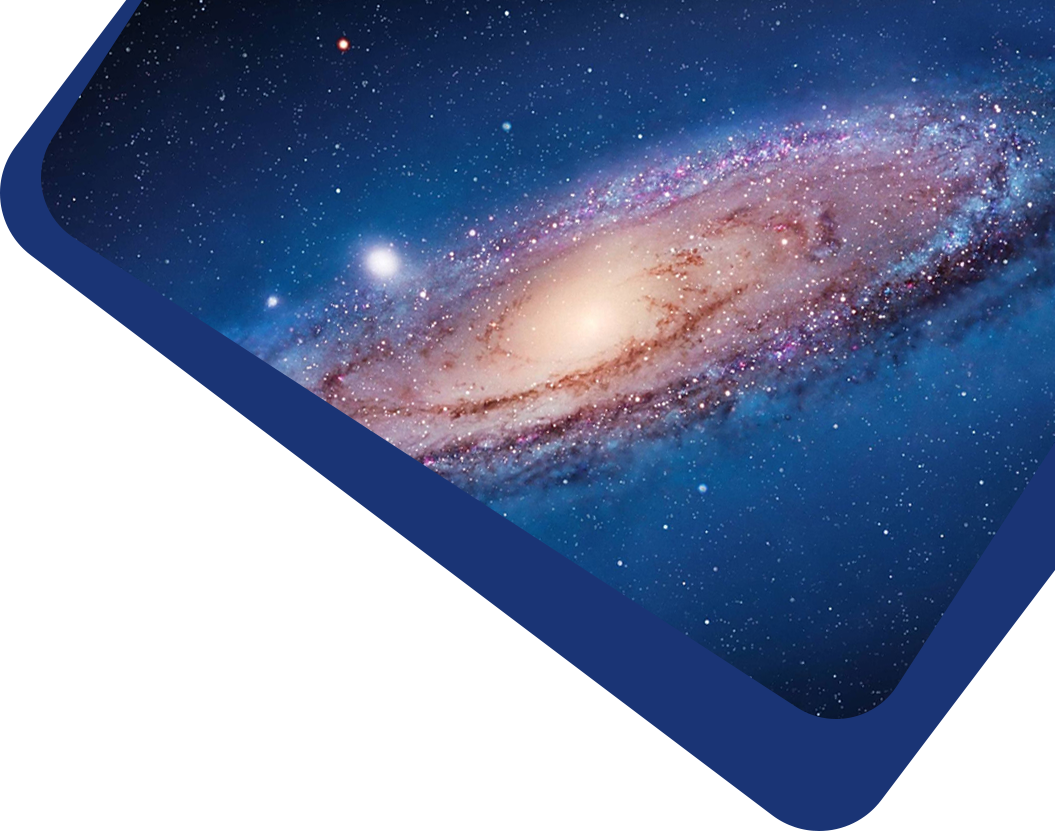Vol 22, No 3

## Solving Surface Deformation of Radio Telescope Antenna by Artificial Neural Network

#### Bo-Yang Wang, Qian Ye, Li Fu, Guo-Xiang Meng, Jin-Qing Wang, Qing-Hui Liu, Zhi-Qiang Shen

Abstract

Abstract Recent investigations have derived the relation between the near-field plane amplitude and the surface deformation of reflector antenna, namely deformation-amplitude equation (DAE), which could be used as a mathematical foundation of antenna surface measurement if an effective numerical algorithm is employed. Traditional algorithms are hard to work directly due to the complex mathematical model. This paper presents a local approximation algorithm based on artificial neural network to solve DAE. The length factor method is used to construct a trial solution for the deformation, which ensures the final solution always satisfies the boundary conditions. To improve the algorithm efficiency, Adam optimizer is employed to train the network parameters. Combining the application of the data normalization method proposed in this paper and a step-based learning rate, a further optimized loss function could be converged quickly. The algorithm proposed in this paper could effectively solve partial differential equations without boundary conditions such as DAE, which at the same time contains the first-order and the second-order partial derivatives, and constant terms. Simulation results show that compared with the original algorithm by Fast Fourier transform, this algorithm is more stable and accurate, which is significant for the antenna measurement method based on DAE.

Keywords

Keywords methods: numerical – telescopes – waves – scattering

Full Text
Refbacks

• There are currently no refbacks.